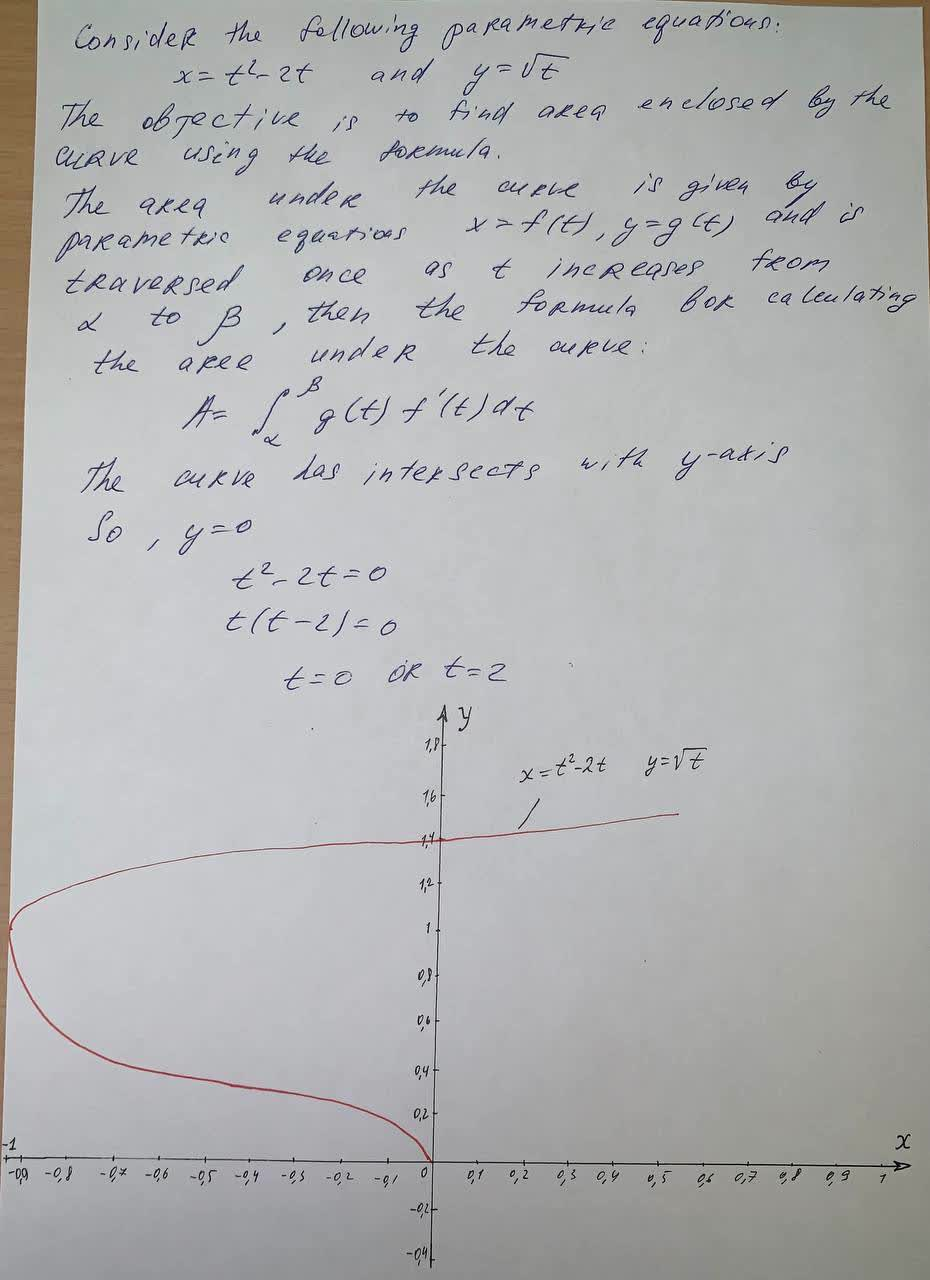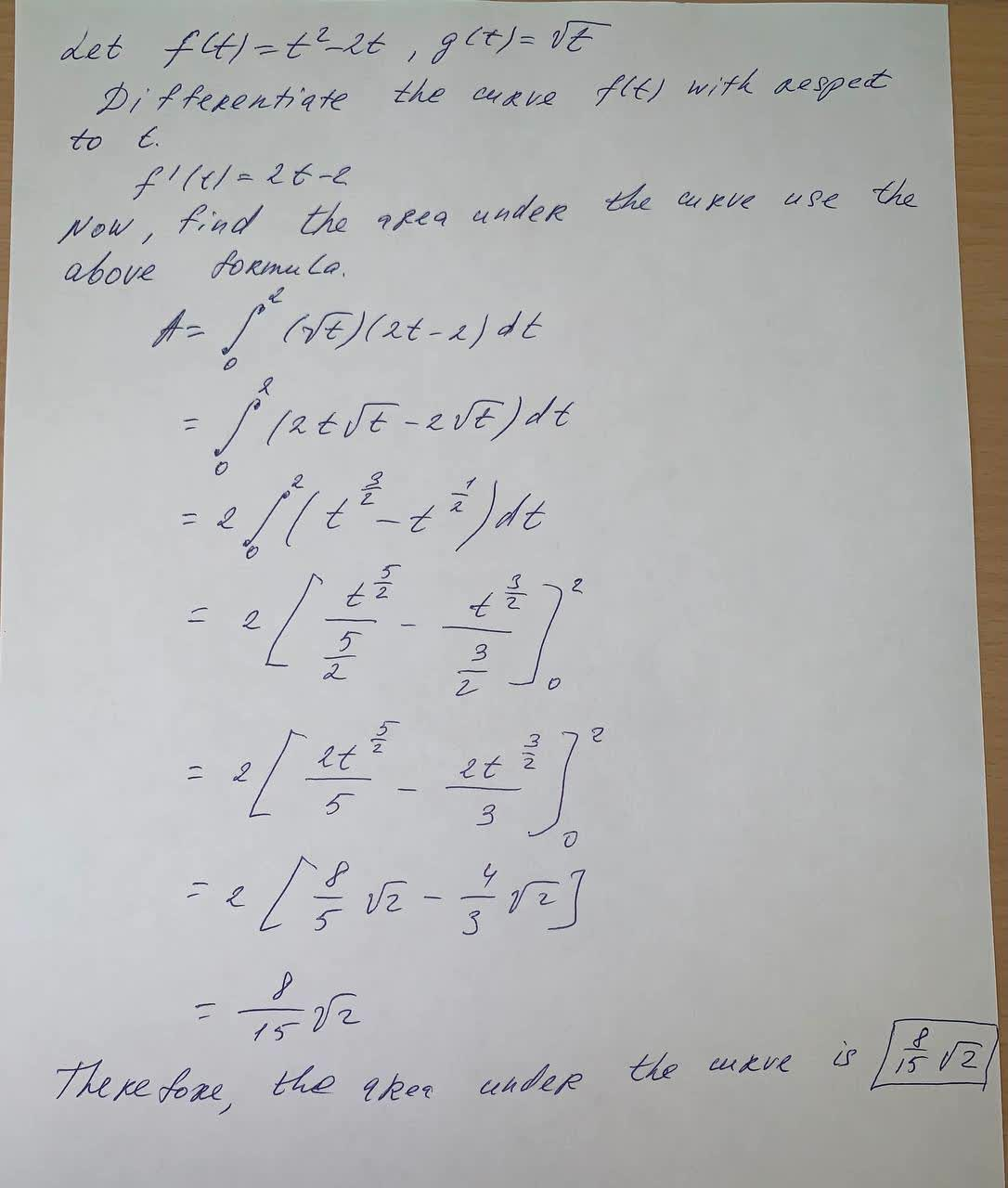Find the area enclosed by the curve x = t^2 - 2t, y = \sqrt{1}t and the y-axis.Globokim8 2021-05-31 Answered
Find the area enclosed by the curve
$$x = t^2 - 2t, y = \sqrt{1}t$$ and the y-axis.

• Questions are typically answered in as fast as 30 minutes

Solve your problem for the price of one coffee

• Math expert for every subject
• Pay only if we can solve itAGRFTr
Hope this helps you: